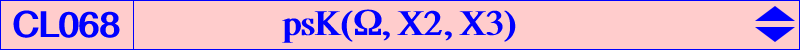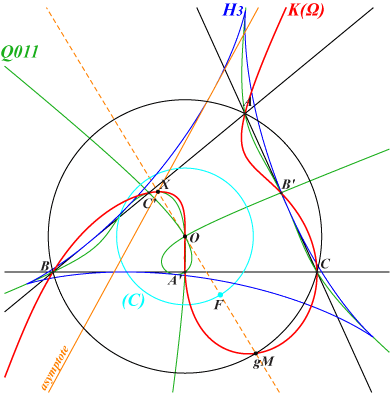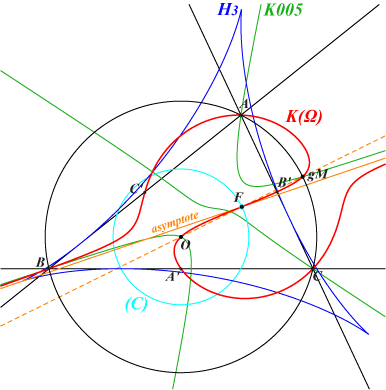Let Ω = p : q : r be a point and K(Ω) the pseudo-pivotal cubic psK(Ω, X2, X3). See Pseudo-Pivotal Cubics and Poristic Triangles, specially Tables 3 and 4. See also the Thomson-Lemoine pencil in Table 50. The equation of K(Ω) is : ∑ p b^2 c^2 y z ((b^2 - c^2) x + a^2 (y - z)) = 0, showing that all these cubics form a net which can be generated by three independent members. It is convenient to choose K(A), K(B), K(C) since these are very simple. Indeed, K(A) is the decomposed cubic, the union of the sidelines AB, AC and the perpendicular bisector of BC. Each cubic K(Ω) contains seven fixed points namely A, B, C, X3 and the midpoints of ABC. Recall that the tangents at A, B, C concur at the pseudo-isopivot Ω. K(Ω) is a pK when it contains G in which case Ω must lie on the Brocard axis. See below. K(Ω) is invariant under two simple transformations, one being the inverse of the other : T1 : M = x : y : z → p y z (x + y - z) (x - y + z) : : , T2 : M = x : y : z → p y z (- p y z + q x z + r x y) : : . *** Other points on K(Ω) : • K(Ω) meets the line at infinity at the same points as pK(X6, atgΩ). The six remaining common points lie on the circum-conic with perspector the X32-isoconjugate of the tripole of the line Ω-X6. This latter conic passes through gtgΩ. • K(Ω) meets the circumcircle at the same points as pK(X6, tgΩ). The three remaining common points lie on the line which is the isogonal transform of the conic above and one of them is the pivot tgΩ. • Apart tgΩ, K(Ω) also contains the Ω-crossconjugate of X(6) which is gatgΩ. Note that the three cubics coincide into K002 when Ω = X6. Now, if mΩ denotes the midpoint of atgΩ, tgΩ (which is actually ctgΩ and also the isogonal conjugate of the cevapoint of X6 and Ω), it can be easily verified that K(Ω) is spK(atgΩ, mΩ) as in CL055 with all the consequences mentioned there. *** Isogonal transform K(Ω)* of K(Ω) K(Ω)* is psK(X6 x gΩ, gΩ, X4) and also spK(tgΩ, ctgΩ). Its pseudo-isopivot is X(6) hence the tangents at A, B, C are the symmedians. When they are distinct i.e. Ω ≠ X6, these cubics K(Ω) and K(Ω)* generate a pencil of circum-cubics which are all spK(P, ctgΩ) for some point P on the line L(Ω) passing through X(2), tgΩ, ctgΩ, atgΩ. Every cubic of this pencil passes through A, B, C, the four foci of the inconic with center ctgΩ and perspector gΩ, and two (not always real) isogonal conjugate points on the line G, ctgΩ hence also on the circum-conic passing through K and gctgΩ (in fact, the cevapoint of K and Ω). The pencil contains : • one and only one pK, namely pK(X6, ctgΩ), • a focal isogonal cubic with singular focus F on (O). F is the isogonal conjugate of the infinite point of the line L(Ω), obviously also on the cubic which is a nK with root the infinite point of the trilinear polar of gΩ. • an equilateral cubic if and only if Ω lies on the Lemoine axis (but these cubics decompose into the line at infinity and a circum-conic) or on the trilinear polar L(X112) of X(112) in which case the cubic is a McCay stelloid. K(Ω) is a member of the pencil studied in Table 50. L(X112) passes through X(i) for i = 6, 25, 51, 154, 159, 161, 184, 206, 232, 1194, 1474, 1495, 1660, 1843, 1915, 1971, 1974, etc. *** More generally, the isoconjugation with pole P transforms K(Ω) into psK(P^2÷Ω, P÷Ω, P÷O) where ÷ denotes a barycentric quotient. In particular, the isotomic transforms of K(Ω) is psK(tΩ, tΩ, X264). Anticomplement aK(Ω) of K(Ω) aK(Ω) is obviously a circum-cubic since K(Ω) contains the midpoints of ABC. It is a pK when Ω lies on the Brocard axis (recall that, in this case, K(Ω) is also a pK, see below) and a psK when Ω lies on the bicevian conic C(X3, X6). In this case, the pseudo-pole lies on K555, the pseudo-pivot lies on the Steiner ellipse, the pseudo-isopivot lies on the line at infinity. Hence, the tangents at A, B, C are parallel. Recall that C(X3, X6) is the locus of the X(3)-Ceva conjugate of every point on the Lemoine axis, and equivalently, the locus of the X(6)-Ceva conjugate of every point on the trilinear polar of X(3), a line passing through X(i) for i = 520, 647, 652, 684, 686, 852, 1459, 1636, 3049, etc. Note that C(X3, X6) is the barycentric product of O and the nine points circle. It contains X(i) for i = 3269, 7117, 9475, 15166, 15167, 20728, 20830, 20975, 22084, 22428, 47405 → 47424. The points X(15166) and X(15167) lie on the Brocard axis and on the Steiner inellipse which is bitangent to C(X3, X6) at these points. The common tangents meet at X(647).Special cubics K(Ω) Pivotal cubics K(Ω) is a pK if and only if it contains X2 hence Ω must lie on the Brocard axis. In this case, these cubics form a pencil and each cubic passes through the seven fixed points above and also X2, X6. Obviously, K(Ω) contains the (real or not) square roots of Ω on the Stammler rectangular hyperbola. gΩ lies on the Kiepert hyperbola and K(Ω) also contains the foci of the inconic with perspector gΩ and center ctgΩ. The following table gives a list of all CTC cubics and also a selection of other cubics that pass through at least seven ETC centers (2, 3, 6 are not repeated) and/or some other interesting points.Ω pK or X(i) on the pK for i = 3 K168 / foci of the orthic inconic 6 K002 15 K341a 16 K341b 32 K177 39 K836 187 K043 / circular cubic 216 K612 511 K357 574 K284 800 K924 1691 K252 2092 K253 3003 K489 3094 K1012 5019 K321 50 50, 186, 323, 11597, 14579 58 27, 58, 86, 2248, 6626, 8044 61 61, 302, 473, 3391, 3392, 10640 62 62, 303, 472, 3366, 3367, 10639 371 371, 492, 1585, 3387, 3388, 10962 372 372, 491, 1586, 3373, 3374, 10960 570 54, 570, 1209, 1594, 2963 577 68, 394, 577, 1147, 6503 1692 114, 230, 460, 1692, 1976 2088 2088, 2433, 5664, 13636, 13722, 15328 3284 30, 265, 1511, 3163, 3284, 11064, 14910, 14919 4258 391, 461, 3635, 4258, 15519 4266 8, 995, 3161, 4266, 5233 5007 251, 428, 3589, 5007, 6292, 15321 5008 597, 1383, 5008, 10301, 15810 5065 1593, 5065, 14379, 14390, 14457, 17811 18591 72, 226, 442, 942, 1214, 18591Circular cubics K(Ω) is circular if and only if Ω lies on the Lemoine axis. In this case, these cubics also form a pencil and each cubic meets the line at infinity again at a real point M = tgΩ and the circumcircle again at gM = gtgΩ. The real asymptote is the Simson line of the antipode of gM on (O) hence it envelopes the Steiner deltoid H3 and it meets K(Ω) again on Q011. The singular focus F of K(Ω) lies on C(X3, R/2) since it is the midpoint of X3-gM. The only pK of the pencil is K043 = K(X187) with focus X(14650). There are three focal cubics in this pencil and one of them is always real. They are obtained when Ω is the barycentric product of X6 and one of the infinite points of the Napoleon cubic K005 or, equivalently, the common points of the Lemoine axis and pK(X1501, X51). The singular foci are the midpoints of the segments joining X3 and the common points of (O) and K005. These three focal cubics are actually central cubics, symmetric about their singular focus.Circular K(Ω) Focal K(Ω)The following table (computed by Peter Moses) gives a list of all CTC circular cubics and also a selection of other cubics together with the points M, F mentioned above. 0 in the second column means Ω is unlisted in the current edition of ETC (2018-05-18).M Ω cubic F X(i) on the cubic for i = 30 1495 K446 12041 3, 4, 30, 74, 133, 1511, 11589, 14993 511 237 K570 12042 3, 32, 98, 132, 511, 5000, 5001, 5403, 5404, 5976, 9467 512 669 3, 99, 512, 5139 513 667 3, 100, 513, 5521 514 649 3, 101, 514, 5190 515 0 3, 102, 515, 10570 516 0 3, 103, 516, 14377, 20622 517 0 3, 56, 104, 517, 1145 518 2223 3, 105, 518, 3513, 3514, 20621 519 902 1, 3, 106, 214, 519, 1319, 15898, 20619 520 0 3, 107, 125, 520 521 1946 3, 11, 108, 521 522 663 3, 109, 522, 20620 523 512 K567 1511 3, 110, 136, 523, 6328, 15454 524 187 K043 14650 2, 3, 6, 67, 111, 187, 468, 524, 1560, 2482, 6593, 10354, 13608, 15899, 15900, 18876 525 647 3, 112, 115, 525 526 14270 3, 476, 526, 16221 527 1055 3, 9, 57, 527, 1155, 2291, 6594 532 0 3, 13, 16, 532, 618, 2380, 3479 533 0 3, 14, 15, 533, 619, 2381, 3480 539 0 3, 128, 539, 2383 542 5191 3, 542, 842, 14357 732 8623 3, 39, 732, 733, 1691, 8290 758 3724 3, 10, 36, 65, 758, 759, 10693 912 0 3, 119, 912, 915, 11517, 18838 916 0 3, 118, 916, 917 924 0 3, 135, 924, 925 971 0 3, 971, 972, 7367 1154 0 3, 5, 186, 1141, 1154, 11597, 18402 1503 0 3, 1297, 1503, 14376 2393 0 3, 25, 206, 858, 2373, 2393, 3455, 5181, 15477 2574 0 3, 1113, 1313, 2574 2575 0 3, 1114, 1312, 2575 2782 0 3, 2698, 2782, 14382 2850 0 3, 2766, 2850, 5520 3307 0 3, 1381, 2446, 3307 3308 0 3, 1382, 2447, 3308 3413 5638 3, 1379, 2028, 3413, 6178 3414 5639 3, 1380, 2029, 3414, 6177 3564 0 3, 114, 1692, 3563, 3564, 6337 3738 8648 3, 2222, 3738, 13999 5663 0 3, 477, 5663, 14385, 18809 6000 0 3, 1294, 6000, 14379 6368 15451 3, 137, 933, 6368 8057 0 3, 122, 1301, 8057 8673 0 3, 127, 1289, 8673 8677 0 3, 1309, 3259, 8677 8681 0 3, 126, 2374, 8681 9019 0 3, 23, 141, 427, 9019, 9076 9028 0 3, 5513, 9028, 9085 9033 9409 3, 1304, 3258, 9033 9517 0 3, 935, 5099, 9517 13754 0 3, 113, 403, 1147, 1300, 13754 15311 0 3, 64, 3184, 5897, 6523, 11744, 12096, 15311 15733 0 3, 55, 3660, 6600, 10427, 15728, 15733 17770 0 3, 58, 6626, 17770, 20666Other remarkable cubics K(Ω) is equilateral if and only if Ω = X(51) corresponding to K026. K(Ω) is a psK+ if and only if Ω lies on a complicated cubic passing through X(51), X(154) corresponding to the cubics K026, K426 which are actually both central cubics. K(Ω) is an unicursal cubic if and only if Ω lies on a very complicated sextic passing through X(55), X(184), X(8041) corresponding to the cubics K259, K009, K1068. The table below presents other listed cubics and a selection of K(Ω) with at least seven ETC centers. Some additional points are given : (∞)Knnn and (O)Knnn denote the infinite points and the points on the circumcircle of Knnn.Ω K(Ω) or X(i) on the cubic for i = / remark Other remarkable points 2 K512 (∞)K410, (O)K184, vertices of 1st Brocard triangle 25 K376 (∞)K004, (O)K006 51 K026 / central stelloid (∞)K003, (O)K005 55 K259 / nodal (∞)pK(X6,X145), (O)K692 56 K1060 (∞)K1044, (O)K1059, foci of the Mandart inellipse 110 K559 (∞)pK(X6,X148), (O)K035 154 K426 / central (∞)pK(X6,X3146), (O)K004 184 K009 / nodal (∞)K006, (O)K003 1400 K1272 (∞)K343, (O)pK(X6,X226) 8041 K1068 / nodal (∞)pK(X6, X7760), (O)pK(X6, X7794) 13366 K569 (∞)K005, (O)pK(X6,X140) 33578 K1110 (∞)pK(X6, aX17810), (O)pK(X6, X17810) 902 1, 3, 106, 214, 519, 1319, 15898, 20619 1193 3, 9, 57, 58, 893, 2092, 3666, 4357, 6626 2260 3, 9, 27, 57, 442, 942, 2160, 5249 2361 1, 3, 21, 36, 80, 860, 4511, 6149 3124 3, 76, 115, 512, 2028, 2029, 3413, 3414, 14384 3271 3, 8, 11, 513, 2446, 2447, 3307, 3308 7113 3, 9, 36, 57, 79, 104, 1333, 2245, 3218, 19302Remark : for any Ω on the line passing through X(6) and X(41), K(Ω) passes through X(9) and X(57) just like K002.